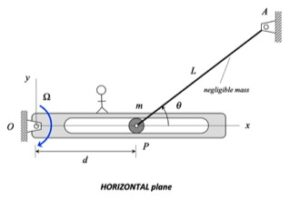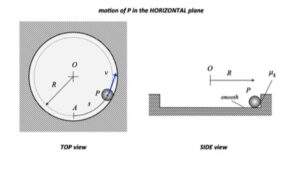# Homework H.4.MHINTS:

STEP 1 - FBD: Draw a SINGLE free body diagram (FBD) of the system of cart + cannon + cannonball.
STEP 2 - Kinetics:  Write down the impulse/momentum equation in the horizontal direction (x-direction) for the the system of cart + cannon + cannonball. Based on the above FBD, is the momentum conserved in the x-direction for that system?
STEP 3 - Kinematics
STEP 4 - Solve. Solve for the velocity of the cart + cannon.

QUESTION: The above analysis allows you to find the answer to the first part of the problem. Unfortunately, it is not useful for the find the answer to the second part of the problem where you want to find the force on the cart/cannon. What do you need to change in the analysis to find this force?

# Homework H.4.NDiscussionShown above is the motion of particle B as it moves through the circular slot in A.

• Consider an FBD of a system made up of A and B together. Since there are no forces acting in the horizontal direction (x-direction), then linear momentum for the system of A+B is conserved in the x-direction. We see evidence of this as we watch the motion above: as the velocity in the x-direction for B increases to the right, then the velocity in of A increases to the left.
• From the above FBD, we can also say that energy is conserved for the system of A+B since there are no external non-conservative forces acting on the system that do work. A consequence of conservation of both energy and linear momentum is that as B reaches its maximum height in the circular slot the total kinetic energy for the system goes to zero since both momentum and kinetic energy was initially zero. You can readily see this in the above animation.

HINTS:

STEP 1 - FBD: Draw a SINGLE free body diagram (FBD) of the system of A+B.
STEP 2 - Kinetics:  Based on the above FBD (and the discussion above), you can use conservation of both energy and linear momentum in the x-direction. Write down both of these equations for the system of A+B.
STEP 3 - Kinematics: At Position 2, the velocity of B is strictly in the x-direction; that is:  vB2 =  vB2x.
STEP 4 - Solve.

# Homework H.4.KDiscussionShown above is the motion of the system made up of blocks A and B, with the velocities of A and B shown in the plots at the top. A is initially moving to the right, and B is initially moving to the left. As A slides on B, friction is generated at the contact of A and B. This friction slows down the motion of A with respect to B to the point where A is no longer moving to the right, and instead A begins moving to the left. You are asked to find the mutual speeds of A and B when sticking occurs.

HINTS:

STEP 1 - FBD: Draw a SINGLE free body diagram (FBD) of the system including Blocks A and B. Note that for this choice of system, the friction force between A and B is internal.
STEP 2 - Kinetics:  From your FBD above, there are no external forces acting on the system in the horizontal direction. What is the implication of this in terms of the linear momentum of the system? (HINT: It says that the linear momentum is conserved for the system of A + B. This becomes your kinetics equation. Note that energy is NOT conserved for this problem due to friction.)
STEP 3 - Kinematics: Note that when slipping ceases between blocks A and B, we have vA2 = vB2.
STEP 4 - Solve. Your final answer should be in terms of vA1 and vB1.

QUESTIONS:

• Should the final answer depend on the coefficient of friction? Does yours?
• Could you have worked out the problem by using two systems: A and B, individually? [Answer: Yes, but it requires more effort since you need to deal with the force of friction.]

__________________________

# Homework H.4.L# Homework H.4.INOTE: All surfaces are smooth. Unfortunately, this information was omitted from the problem statement.

______________________

HINTS

STEP 1 - FBD: Draw a SINGLE free body diagram (FBD) of the system including Block A, Block B and the rigid bar. From this, determine which forces do non-conservative work on this system, if any.
STEP 2 - Kinetics:  Write down the work/energy equation.
STEP 3 - Kinematics: Consider the location of the instant center for bar AB. This will be the key to the kinematics that you need to solve this problem.
STEP 4 - Solve

__________________________

# Homework H.4.JHINTS

STEP 1 - FBD: Draw a SINGLE free body diagram (FBD) of the system including Block A, Block B and the cable. From this, determine which forces do work on this system.
STEP 2 - Kinetics:  Write down the work/energy equation. Determine the work done by the forces that you identified  above in STEP 1.
STEP 3 - Kinematics: Review the constrained motion kinematics from Section 1.D of the course lecture book. To this end, you will write down an expression for the length of the cable in terms of sA, sB and constants. Differentiate this expression to relate the speeds of A and B.
STEP 4 - Solve

__________________________

# Homework H.4.G# Homework H.4.H